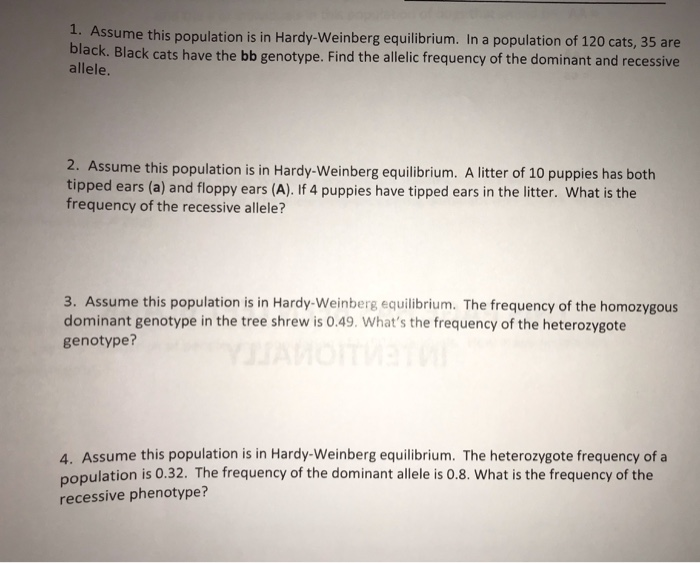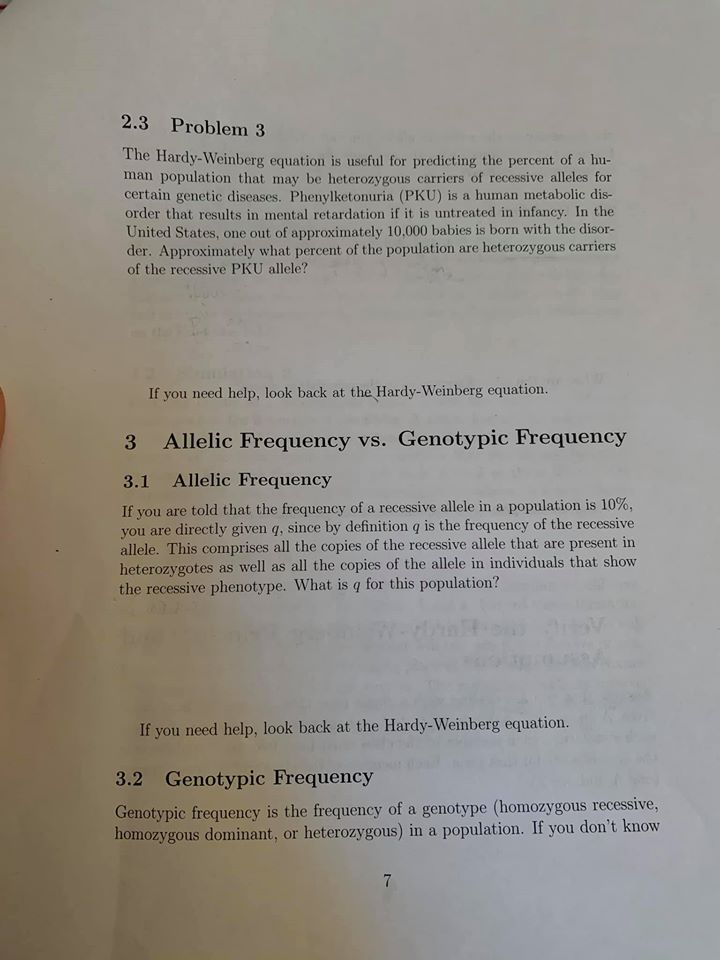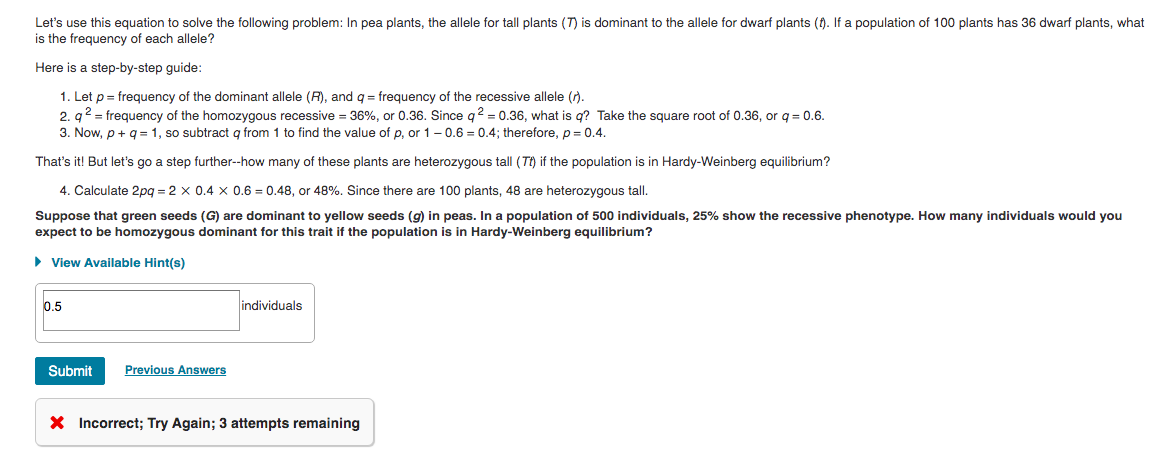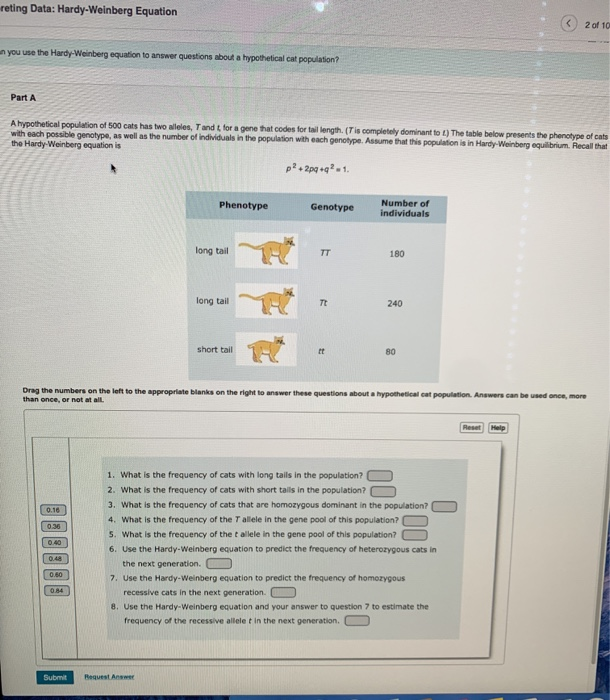Question

# You are a population geneticist studying Gene B in a population that is in Hardy-Weinberg equilibrium....

You are a population geneticist studying Gene B in a population that is in Hardy-Weinberg equilibrium. You sample a population to determine the genotypes at Gene B. You find that the freuency of homozygous recessive genotypes (bb) is 0.10. What would be the frequency of the dominant allele (B) in this population?

A. 0.84

B. 0.4

C. 0.36

D. 0.6

E. 0.48

The frequency of the dominant allele (B) in this population is............................0.68 or 68%

• homozygous recessive genotype................bb = 0.10 or 10%
• The genotype frequency of bb is 10% , that is q2 = 10%
• q2 = 10% or 0.1
• then q = 0.31
• then the allelic frequency of recessive homozyogus "a" is .........31%
• if q = 0.31
• p +q =1, then p =1- q
• p = 1-0.31 = 0.69
• p = 69%
• if p = 69%, q = 31%
• then p2+2pq+q2 = 1
• (0.69)2 + 2 (0.69)(0.31) + (0.31)2 = 1
• 0.4761+0.4278+ 0.0961 =1
• The genotypic frequency of homozygous dominant allele = p2 =47.6%
• then p = p2+1/2 (2pq) = 0.476+1/2(0.4278) = 0.6899
• p or dominant allele "B" phenotypic frequency = 0.6899
• then q equals to 1-p = 1-0.6899 = 0.31
• 68 % of the population show dominant phenotype.
• 31 % of the population show recessive phenotype.

#### Earn Coins

Coins can be redeemed for fabulous gifts.

Similar Homework Help Questions
• ### Evolution 1. Assume this population is in Hardy-Weinberg equilibrium. In a population of 120 cats, 35...Evolution 1. Assume this population is in Hardy-Weinberg equilibrium. In a population of 120 cats, 35 are black. Black cats have the bb genotype. Find the allelic frequency of the dominant and recessive allele. 2. Assume this population is in Hardy-Weinberg equilibrium. A litter of 10 puppies has both tipped ears (a) and floppy ears (A). If 4 puppies have tipped ears in the litter. What is the frequency of the recessive allele? 3. Assume this population is in Hardy-Weinberg...

• ### The Hardy-Weinberg principle and its equations predict that frequencies of alleles and genotypes remain constant from generation to generation in populations that are not evolving

.1. The Hardy-Weinberg principle and its equations predict that frequencies of alleles and genotypes remain constant from generation to generation in populations that are not evolving. What five conditions does this prediction assume to be true about such a population? a._______  b._______  c._______  d._______  e._______  2. Before beginning the activity, answer the following general Hardy-Weinberg problems for practice (assume that the population is at Hardy-Weinberg equilibrium).a. If the frequency of a recessive allele is 0.3, what is the frequency of the dominant...

• ### 2.3 Problem 3 The Hardy-Weinberg equation is useful for predicting the percent of a hu- man...2.3 Problem 3 The Hardy-Weinberg equation is useful for predicting the percent of a hu- man population that may be heterozygous carriers of recessive alleles for certain genetic diseases. Phenylketonuria (PKU) is a human metabolic dis- order that results in mental retardation if it is untreated in infancy. In the United States, one out of approximately 10.000 babies is born with the disor- der. Approximately what percent of the population are heterozygous carriers of the recessive PKU allele? If you...

• ### 1.)If the population frequencies of two alleles at a locus are B = 0.5 and b...

1.)If the population frequencies of two alleles at a locus are B = 0.5 and b = 0.5, what is onepossible set of frequencies for the three resulting genotypes that would NOT reflect Hardy- Weinberg equilibrium? 2.)In a population that is in Hardy-Weinberg equilibrium, the frequency of the homozygous recessive genotype is 0.09. What is the frequency of individuals that are homozygous for the dominant allele? 3.)In humans, Rh-positive individuals have the Rh antigen on their red blood cells, while...

• ### Equations: p + q=1 p2 + 2(p)(q) + q2 1) In a population with Hardy-Weinberg equilibrium,...

Equations: p + q=1 p2 + 2(p)(q) + q2 1) In a population with Hardy-Weinberg equilibrium, the frequency of a dominant allele for a gene is 85% (0.85) while the frequency of the recessive allele for the same gene is 15% (0.15). 0.0005 0.013 0.675 0.723 0.255 0.50 0.023 2) proportion of individuals will be homozygous recessive? 0.0005 0.013 0.675 0.723 0.255 0.50 0.023 3) proportion of individuals will be homozygous dominant? 0.0005 0.723 0.013 0.675 0.255 0.50 0.023 4)...

• ### Let's use this equation to solve the following problem: In pea plants, the allele for tall...Let's use this equation to solve the following problem: In pea plants, the allele for tall plants (T) is dominant to the allele for dwarf plants (1). If a population of 100 plants has 36 dwarf plants, what is the frequency of each allele? Here is a step-by-step guide: 1. Let p = frequency of the dominant allele (R), and q = frequency of the recessive allele (1). 2.92 = frequency of the homozygous recessive = 36%, or 0.36. Since...

• ### 1. Suppose a population of organisms is in Hardy–Weinberg equilibrium. In the population, a gene has...

1. Suppose a population of organisms is in Hardy–Weinberg equilibrium. In the population, a gene has two alleles, B and b. If the frequency of B is 0.72 and the frequency of b is 0.28, what are the expected genotype frequencies of BB, Bb, and bb? Calculate each frequency to two decimal places.

• ### questions g,h, i, j. thank you Use the Hardy-Weinberg theorem for populations in equilibrium to answer the Suppose a gene has two alleles, B and b, with B dominant. If the frequency of the allele...questions g,h, i, j. thank you Use the Hardy-Weinberg theorem for populations in equilibrium to answer the Suppose a gene has two alleles, B and b, with B dominant. If the frequency of the allele B a. What percentage of the population display the phenotype B? b. What percentage of the population are carriers for the recessive c. What percentage of the population display the recessive phenotype? following questions. is 0.7, es alleleb? allele b? If 1 6% of people...

• ### (Hardy Weinberg Equation) In a population of rabbits, there are 423 A1A1, 1484 A2A2, and 1661...

(Hardy Weinberg Equation) In a population of rabbits, there are 423 A1A1, 1484 A2A2, and 1661 A1A2 individuals. If the enviornment changes so that the homozygous recessive genotype suffers a reduction of fitness where it's fitness is now 0.59 , but the other genotypes are unaffected, what will be the frequency of the dominant allele in the NEXT generation?

• ### reting Data: Hardy-Weinberg Equation 2 of 10 you use the Hardy Weinberg equation to answer questions...reting Data: Hardy-Weinberg Equation 2 of 10 you use the Hardy Weinberg equation to answer questions about a hypotheticalcat population Part A A hypothetical population of 500 cats has two wees, Tandt for a gene that codes for tail length (Tis completely dominantot) The table below presents the phenotype of cats with each possible genotype, as well as the number of individuals in the population with each genotype. Assume that this population is in Hardy-Weinberg equilibrium Recall that the Hardy...